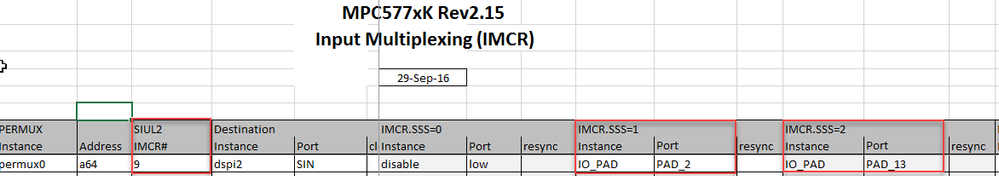## SPI 0&SPI 2

cancel
Showing results for
Did you mean:

## SPI 0&SPI 2

714 ViewsContributor III

I want to use SPI0 and SPI2 are set to the MASTER mode to configure two chips.My SPIO configuration no problem, but SPI2 can not read the data. I can see the data sent and returned on my oscilloscope. But the data out of the register can not be written into。ＭＣＵ　ｉｓ　５７７５Ｋ　．

IO：    //SPIO CLK
SIUL2.MSCR[SPI0_SCK_MSCR_NB].R = MSCR_OUTPUT|SPI0_SCK_MSCR_SSS;
SIUL2.MSCR[SPI0_SCK_MSCR_NB].B.SRC = 3;    /* Full strength slew rate */
//SPIO SOUT
SIUL2.MSCR[SPI0_SOUT_MSCR_NB].R = MSCR_OUTPUT|SPI0_SOUT_MSCR_SSS;
SIUL2.MSCR[SPI0_SOUT_MSCR_NB].B.SRC = 3;    /* Full strength slew rate */
//SPIO SIN
SIUL2.MSCR[SPI0_SIN_MSCR_NB].R = MSCR_INPUT;
//SPIO CS
SIUL2.MSCR[SPI0_CS0_MSCR_NB].R = MSCR_OUTPUT|SPI0_CS0_MSCR_SSS;
SIUL2.MSCR[SPI0_CS0_MSCR_NB].B.SRC = 3;    /* Full strength slew rate */

//SPI2 CLK
SIUL2.MSCR[SPI2_SCK_MSCR_NB].R = MSCR_OUTPUT|SPI2_SCK_MSCR_SSS;//10
SIUL2.MSCR[SPI2_SCK_MSCR_NB].B.SRC = 3;
//SPI2 SOUT
SIUL2.MSCR[SPI2_SOUT_MSCR_NB].R = MSCR_OUTPUT|SPI2_SOUT_MSCR_SSS;//12
SIUL2.MSCR[SPI2_SOUT_MSCR_NB].B.SRC = 3;
//SPI2 SIN
SIUL2.MSCR[SPI2_SIN_MSCR_NB].R = MSCR_INPUT;//13
//SPI2 CS
SIUL2.MSCR[SPI2_CS0_MSCR_NB].R = MSCR_OUTPUT|SPI2_CS0_MSCR_SSS;//10
SIUL2.MSCR[SPI2_CS0_MSCR_NB].B.SRC = 3;

void SPI0_Init(void)
{
SPI_0.MCR.R = 0x80010001;            /* Configure SPI as master */

SPI_0.MODE.CTAR.B.DBR     = 0;    /* 50/50 duty cycle */
SPI_0.MODE.CTAR.B.FMSZ     = 15;    /* 16 bits per frame */
SPI_0.MODE.CTAR.B.CPOL     = 0;    /* Inactive state value of SCK is low */
SPI_0.MODE.CTAR.B.CPHA     = 0;    /* Data captured and then changed */
SPI_0.MODE.CTAR.B.LSBFE     = 0;    /* MSB first */

/* Tcsc = 1/fp * PCSSCK * CSSCK */
SPI_0.MODE.CTAR.B.PCSSCK = 3;    /* 7, PCS to SCK delay prescaler */
SPI_0.MODE.CTAR.B.CSSCK     = 0;    /* 2, PCS to SCK delay scaler */

/* Tasc = 1/fp * PASC * ASC */
SPI_0.MODE.CTAR.B.PASC     = 0;    /* 1, After SCK delay prescaler */
SPI_0.MODE.CTAR.B.ASC     = 0;    /* 2, After SCL delay scaler */

/* Tdt = 1/fp * PDT * DT */
SPI_0.MODE.CTAR.B.PDT     = 3;    /* 7, Delay after transfer prescaler */
SPI_0.MODE.CTAR.B.DT     = 0;    /* 2, Delay after transfer scaler */

/* baud rate = fp/PBR * (1+DBR)/BR */
SPI_0.MODE.CTAR.B.PBR     = 3;    /* 7, Baud rate prescaler */
SPI_0.MODE.CTAR.B.BR     = 0;    /* 2, Baud rate scaler */

SPI_0.MCR.B.HALT = 0x0;                /* Exit HALT mode: go from STOPPED to RUNNING state*/

}

void SPI2_Init(void)
{
SPI_2.MCR.R = 0x80010001;            /* Configure SPI as master */

SPI_2.MODE.CTAR.B.DBR     = 0;    /* 50/50 duty cycle */
SPI_2.MODE.CTAR.B.FMSZ     = 15;    /* 16 bits per frame */
SPI_2.MODE.CTAR.B.CPOL     = 0;    /* Inactive state value of SCK is low */
SPI_2.MODE.CTAR.B.CPHA     = 0;    /* Data captured and then changed */
SPI_2.MODE.CTAR.B.LSBFE     = 0;    /* MSB first */

/* Tcsc = 1/fp * PCSSCK * CSSCK */
SPI_2.MODE.CTAR.B.PCSSCK = 3;    /* 7, PCS to SCK delay prescaler */
SPI_2.MODE.CTAR.B.CSSCK     = 0;    /* 2, PCS to SCK delay scaler */

/* Tasc = 1/fp * PASC * ASC */
SPI_2.MODE.CTAR.B.PASC     = 0;    /* 1, After SCK delay prescaler */
SPI_2.MODE.CTAR.B.ASC     = 0;    /* 2, After SCL delay scaler */

/* Tdt = 1/fp * PDT * DT */
SPI_2.MODE.CTAR.B.PDT     = 3;    /* 7, Delay after transfer prescaler */
SPI_2.MODE.CTAR.B.DT     = 0;    /* 2, Delay after transfer scaler */

/* baud rate = fp/PBR * (1+DBR)/BR */
SPI_2.MODE.CTAR.B.PBR     = 3;    /* 7, Baud rate prescaler */
SPI_2.MODE.CTAR.B.BR     = 0;    /* 2, Baud rate scaler */

SPI_2.MCR.B.HALT = 0x0;                /* Exit HALT mode: go from STOPPED to RUNNING state*/

}

void SPI0_Transmit(SPI_CS csn, uint16_t sout, uint16_t *sin)
{
SPI_0.PUSHR.PUSHR.R = 0x08000000 | (uint32_t)csn << 16 | (uint32_t)sout;
while (SPI_0.SR.B.TCF == 0);
SPI_0.SR.B.TCF = 1;
*sin = SPI_0.POPR.R;
}

void SPI2_Transmit(SPI_CS csn, uint16_t sout, uint16_t *sin)
{
SPI_2.PUSHR.PUSHR.R = 0x08000000 | (uint32_t)csn << 16 | (uint32_t)sout;
while (SPI_2.SR.B.TCF == 0);
SPI_2.SR.B.TCF = 1;
*sin = SPI_2.POPR.R;　　　　　　　　　　　　　　　　　／／The problem is here
}

Tags (2)
2 Replies
342 ViewsNXP TechSupport

Hi,

For the SPI2 SIN you also need to configure the IMCR register depending on used pad.

SIUL2.IMCR.B.SSS = 1;    /* SPI2 SIN: connected to pad2 */

SIUL2.IMCR.B.SSS = 2;    /* SPI2 SIN: connected to pad13 */BR, Petr

342 ViewsContributor III

//SPI2 CLK
SIUL2.MSCR[SPI2_SCK_MSCR_NB].R = MSCR_OUTPUT|SPI2_SCK_MSCR_SSS;//11
SIUL2.MSCR[SPI2_SCK_MSCR_NB].B.SRC = 3;
//SPI2 SOUT
SIUL2.MSCR[SPI2_SOUT_MSCR_NB].R = MSCR_OUTPUT|SPI2_SOUT_MSCR_SSS;//12
SIUL2.MSCR[SPI2_SOUT_MSCR_NB].B.SRC = 3;
//SPI2 SIN
SIUL2.MSCR[SPI2_SIN_MSCR_NB].R = MSCR_INPUT;//13
//SPI2 CS
SIUL2.MSCR[SPI2_CS0_MSCR_NB].R = MSCR_OUTPUT|SPI2_CS0_MSCR_SSS;//10
SIUL2.MSCR[SPI2_CS0_MSCR_NB].B.SRC = 3;